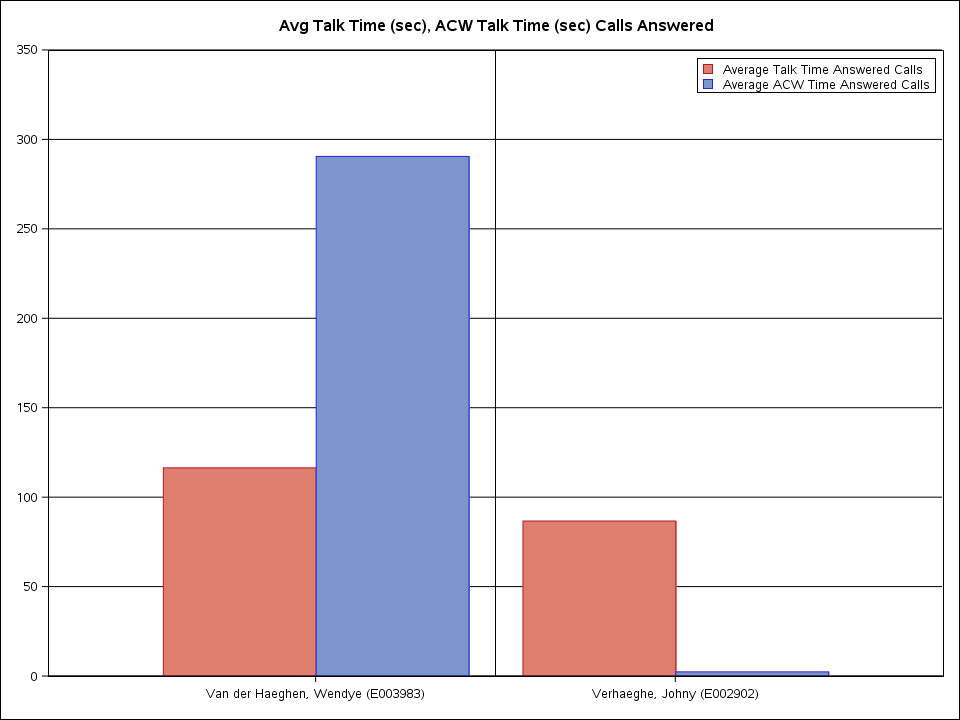## Bar Chart

I want to create a bar chart as follows with the following data. I knew that we can accomplish this using proc sgplot but I'm not certain to create a chart for each observation. Appreciate if someone of you help me here.

Data preparation:

``````data test;
name='Van der Haeghen, Wendye (E003983)';
name='Verhaeghe, Johny (E002902)';
;
run;

set test;
run;``````

Dataset:

 name Avg_Talk_Time_Answered_Calls Avg_ACW_Time_Answered_Calls Van der Haeghen, Wendye (E003983) 1.163.953.488 2.904.883.721 Verhaeghe, Johny (E002902) 8.666.666.667 2.333.333.333

1 ACCEPTED SOLUTION

Accepted Solutions

## Re: Bar Chart

``````data test;
name='Van der Haeghen, Wendye (E003983)';
name='Verhaeghe, Johny (E002902)';
;
run;

set test;
run;

data plot;
set temp;
length category \$100.;
run;

ods graphics / reset width=10in;
title 'Avg Talk Time (sec), ACW Talk Time (sec) Calls Answered';
proc sgplot data=plot;
vbar name / response=time group=category groupdisplay=cluster grouporder=descending;
keylegend / position=topright location=inside down=2 title='';
refline 'Van der Haeghen, Wendye (E003983)' / axis=x discreteoffset=0.5 lineattrs=(color=black pattern=1);
yaxis min=0 max=350 values=(0 to 350 by 50) grid gridattrs=(color=black pattern=solid) display=(nolabel) offsetmin=0 offsetmax=0;
xaxis display=(nolabel) offsetmin=0.3 offsetmax=0.3;
run;``````18 REPLIES 18

## Re: Bar Chart

SGPLOT only allows clustered or stacked bar charts. Neither of those is what you show as the desired output where person #1 is on the left, and person #2 is on the right.

If you want clustered or stacked bar charts, then yes this is possible.

--
Paige Miller

## Re: Bar Chart

As I said, SGPLOT cannot produce the output you asked for.

--
Paige Miller

## Re: Bar Chart

@Babloo wrote:

Could you please provide some example data in the form of a data step so we can actually test code with data?

OR reference a SAS supplied data set in the SASHELP library that may be able to demonstrate similar data types and desired behavior for that data set.

You desired output picture makes me suspect SGPANEL might be possible but without data to test I'm afraid that's as far as I want to go.

## Re: Bar Chart

The data is in their code in the original post. I made the graph in SGPLOT in another reply if you want to see it.

## Re: Bar Chart

Thank you for the code. Is there any way that you can produce a similar
chart in Proc report or proc tabulate?

## Re: Bar Chart

@Babloo SGPLOT is included in SAS BASE, you should be able to run @JeffMeyers code without issues.

## Re: Bar Chart

I am afraid I would have to disagree with you there.  Whilst the base plot would not do what is required, there is nothing to say that you cannot overplot each.  So essentially you would create a four group formatted value:

Wendy, Average talk time = 1

Wendy, Average ACW = 2

Johny, Average talk time = 4

Johny, Average ACW = 5

Then you would graph this based on a non displayed X Axis, with values = 0,1,2,3,4,5,6

At position x=3 you would draw a refence line.

You woul dneed to fiddle a bit with the legend, maybe just text draw the two bits.

There are thousands of examples of all types of graphs - bookmark that page as its the best reference for graphing.

## Re: Bar Chart

With a different data structure, proc gchart (as used by the bar chart wizard in EG) can produce something similar:

``````data have;
infile cards dsd dlm=' ';
input person :\$50. type :\$20. value :commax20.;
cards;
"Van der Haeghen, Wendye (E003983)" avg_talk_time 116,3953488
"Van der Haeghen, Wendye (E003983)" avg_acw_time 290,4883721
"Verhaeghe, Johny (E002902)" avg_talk_time 86,66666667
"Verhaeghe, Johny (E002902)" avg_acw_time 2,333333333
;
run;

PROC GCHART DATA=have
;
VBAR
person
/
SUMVAR=value
GROUP=type
CLIPREF
SPACE=0
FRAME  TYPE=SUM
COUTLINE=BLACK
RAXIS=AXIS1
MAXIS=AXIS2
PATTERNID=MIDPOINT
;
run;``````

In the wizard, the diagram column is person, bars are grouped by type, and value is used as "sum of".

## Re: Bar Chart

Thank you for the code. Is there any way that we try with proc freq except the coloring part? I just noticed that I do not licensed for graphical procedures.

## Re: Bar Chart

SGPLOT does not require a SAS/GRAPH license and is more powerful.

@Babloo wrote:

Thank you for the code. Is there any way that we try with proc freq except the coloring part? I just noticed that I do not licensed for graphical procedures.

## Re: Bar Chart

I received the following error message after executing the proc sgplot code. Is that not a license issue?

``````53         proc sgplot data=plot;
ERROR: The SAS/GRAPH product with which SGPLOT (2) is associated is either not licensed for your system or the product license has
ERROR: Bad product ID for procedure SGPLOT.``````

## Re: Bar Chart

``````data test;
name='Van der Haeghen, Wendye (E003983)';
name='Verhaeghe, Johny (E002902)';
;
run;

set test;
run;

data plot;
set temp;
length category \$100.;
run;

ods graphics / reset width=10in;
title 'Avg Talk Time (sec), ACW Talk Time (sec) Calls Answered';
proc sgplot data=plot;
vbar name / response=time group=category groupdisplay=cluster grouporder=descending;
keylegend / position=topright location=inside down=2 title='';
refline 'Van der Haeghen, Wendye (E003983)' / axis=x discreteoffset=0.5 lineattrs=(color=black pattern=1);
yaxis min=0 max=350 values=(0 to 350 by 50) grid gridattrs=(color=black pattern=solid) display=(nolabel) offsetmin=0 offsetmax=0;
xaxis display=(nolabel) offsetmin=0.3 offsetmax=0.3;
run;``````Discussion stats
• 18 replies
• 1360 views
• 1 like
• 8 in conversation